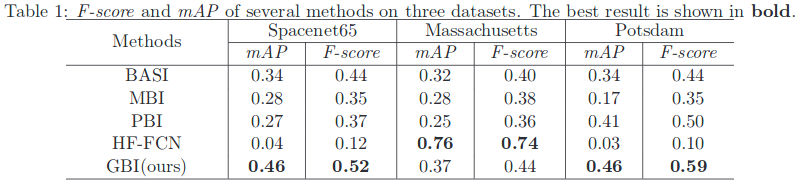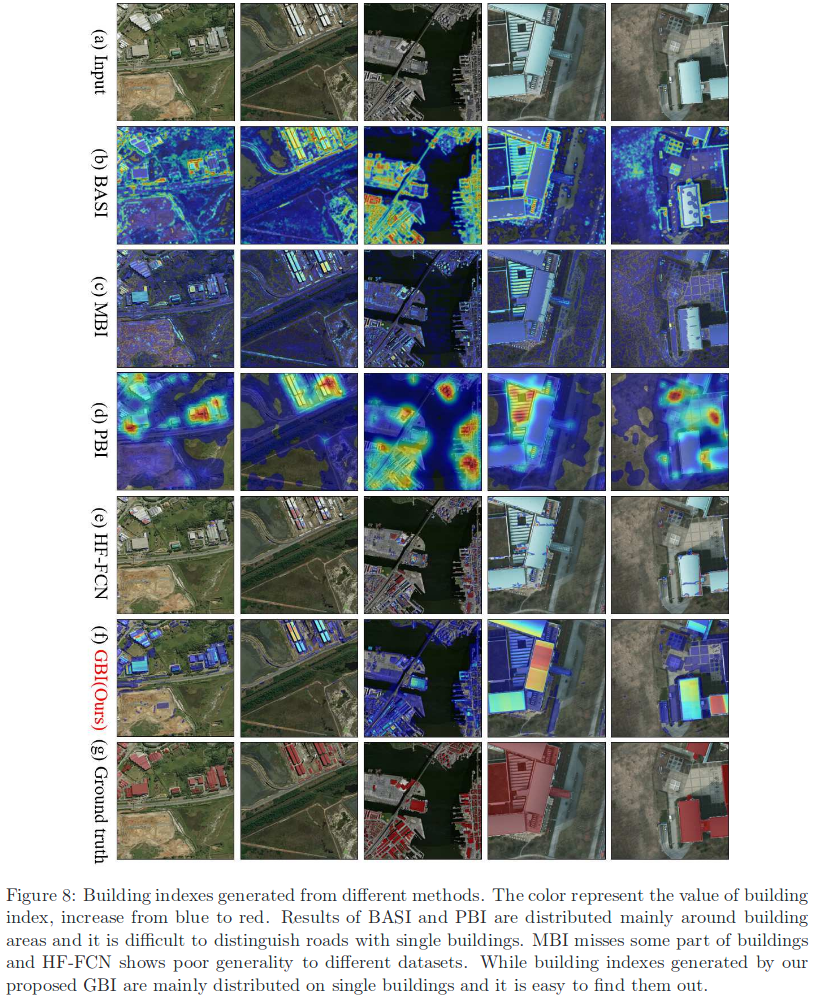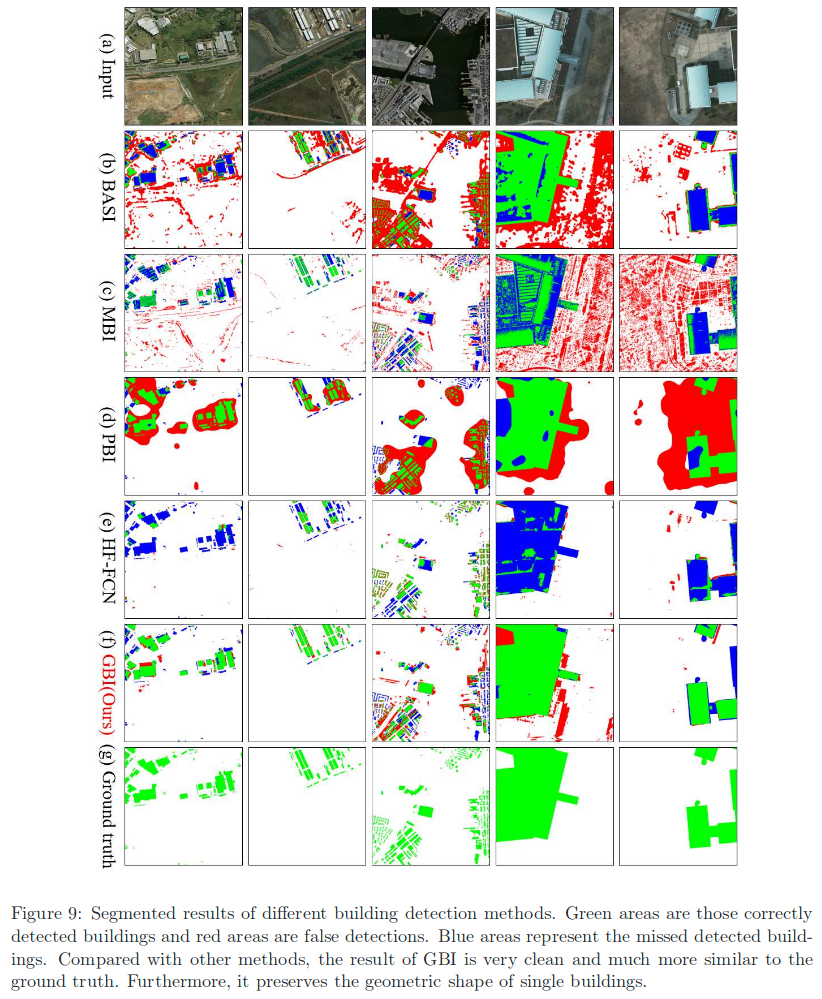• The code of ASJ: https://github.com/cherubicXN/anisotropic-scale-junction-detector
• The model of HF-FCN: https://github.com/tczuo/HF-FCN-for-Robust-Building-Extraction
• Results: http://captain.whu.edu.cn/project/geosay.html

# Summary

Automatic extraction of buildings in remote sensing images is an important task in different fields like urban planning, navigation and more. This paper addresses the problem of buildings extraction in very high-spatial-resolution (VHSR) remote sensing (RS) images. This paper proposes a geometric building index (GBI) for accurate building extraction, by computing the geometric saliency from VHSR-RS images. Given an image, the geometric saliency is derived from a mid-level geometric representations based on the junctions that can locally describe geometrical structures of images. Additionally, GBI preserves both the exact position and accurate shape of single buildings compared to existing methods.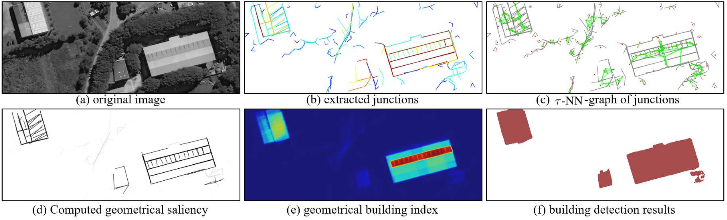# Method

## Junction-based representation of images

Detecting junctions in an image is to find all the local structures j, modeled by the template and estimate their parameters. They used the a-contrario junction detector (ACJ) where they assumed that the scale of branches are identical. With ACJ detector, from the intersected point of a junction, they need to define a measurement to judge whether there exists junction or not and find those branches. (ASJ: Anisotropic Scale Junction Detection and Matching)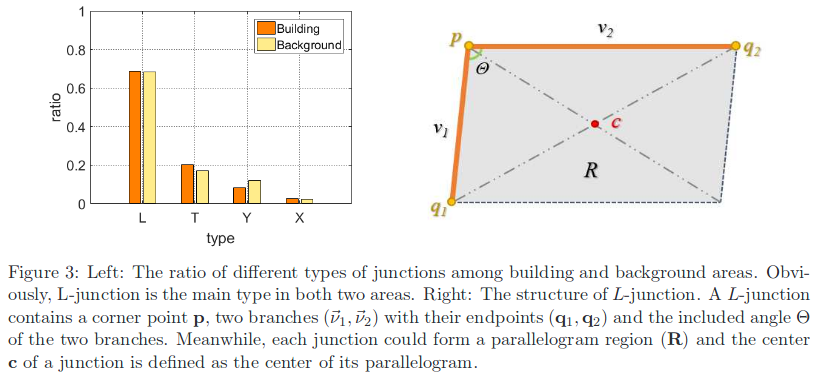## Statistics of junctions in VHR RS images

One of the observations made is that, in RS images, the statistics of junctions should be different on buildings and background. As junctions are detected along areas with high gradients, they are likely to be found around corners of buildings. Buildings are typical man-made objects and their shape are usually very regular or more precisely, rectangular. Thus, the included angles $$\theta$$ of L-junctions will also have special distribution when they are located along buildings. To verify this supposition, they calculated the distributions of L-junctions included angles among different regions from the Spacenet65 dataset. One can find that junctions included angles are really close to $$\pi/2$$ in the building area and have a large difference towards junctions among background area. In building area, angles of junctions are highly concentrated in interval [$$\pi / 3$$, $$2\pi / 3$$]. While in the background area, distribution around $$\pi/2$$ has less contrast to other intervals. Such distributions can help us to distinguish junctions around buildings from other objects, and can be used as a prior in the detection of buildings.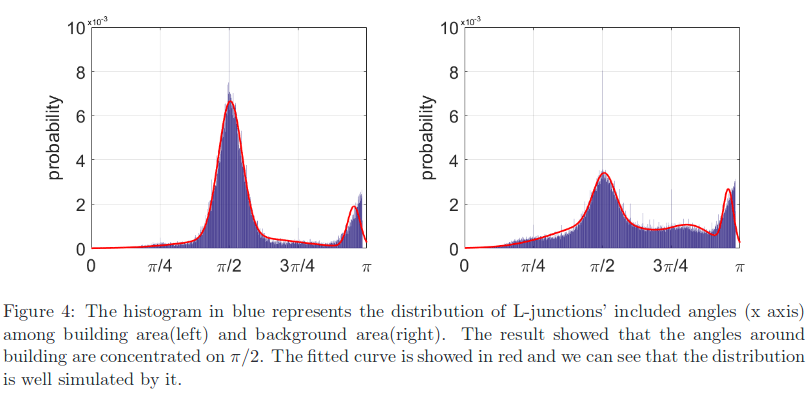## GeoSay: from junctions to building index

Given an image, all detected junctions J can be divided into two subsets,i.e., $$J=J_{B}\cup J_{\bar{B}}$$, where $$J_{B}$$ mean inside the buildings and $$J_{\bar{B}}$$ outside the buildings. For a junction j with its parametric description $$\theta_{j}=\{c, \vec{\nu}_{1}, \vec{\nu}_{2}, \beta, \rho\}$$, the posterior probability $$P(j\in J_{B}|\theta_{j})$$, measuring the possibility of the event that ajunction j is inside buildings, can be derived by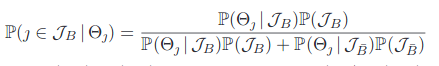Where the prior probabilities $$P(J_{B})$$, $$P(J_{\bar{B}})$$ and the likelihoods $$P(\theta_{j}\|J_{B})$$, $$P(\theta_{j}\|J_{\bar{B}})$$ can be estimated from a given dataset of buildings, e.g. the Spacenet65 dataset, based on the fitted GMM model.

By combining the significance parameter and included angle of a single junction, the first-order geometric saliency $$g^{(1)}_{j}$$ of a junction j can be computed as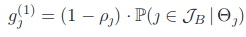Which indicates the degree of a single junction locating along buildings.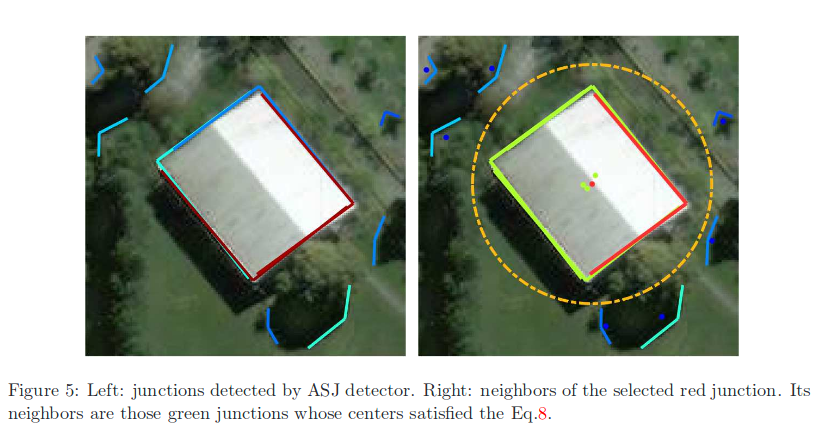An example of junctions and neighboring junctions is displayed in Fig.5, where green points are the centers of junctions inside the $$\tho-NN$$ of the junction with location center in red. Based on neighboring junctions, the pair-wise geometric saliency of a junction $$j$$ is defined as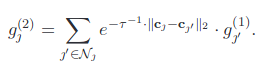Geometric building index, attempts to associate each pixel p with a saliency measuring the possibility of the pixel belonging to buildings, which is the summation of saliency inside parallelogram of all junctions.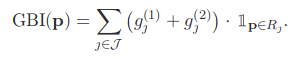# Results